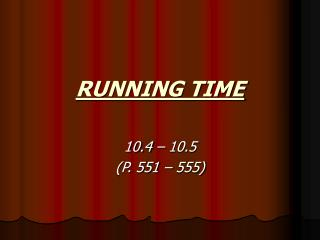DownloadDownload PresentationRUNNING TIME

# RUNNING TIME

Télécharger la présentation## RUNNING TIME

- - - - - - - - - - - - - - - - - - - - - - - - - - - E N D - - - - - - - - - - - - - - - - - - - - - - - - - - -
##### Presentation Transcript

1. RUNNING TIME 10.4 – 10.5 (P. 551 – 555)

2. RUNNING TIME • analysis of algorithms involves analyzing their effectiveness • need way of "guessing" how fast the algorithm will run without actually programming it and running it • to determine the speed, you must consider running time regardless of size of data set used for input • this is known as time complexity, and we often want to consider the worst case

3. What is Big-O Notation • goal is to identify the dominant factor in determining the execution time of our algorithm • in most cases, this is some function of the size of the input data, denoted by n • goal then is to see how the execution time varies with size of data set • we consider the steps of an algorithm may be: • constant (will not change regardless of n) example: x = initvalue; x[n-1] = initvalue; • a function of n (changes with input size) example: for (int i = 1; i < n; i++) x[i] = x[i-1] * factor; (number of steps executed changes with size of n)

4. BIG-O Notation So, How Does this Relate to Big-O? • Big-O is the notation used for analyzing the upper bound of an algorithm • in the above examples, you can easily count the steps, but this is not always the case (sometimes it is almost impossible to tell how many steps will be taken) • thus, we usually talk about best case, average case or worst cast; • Big-O most often refers to worst case

5. BIG-O Notation (Definition): • f(n) = O(g(n)) if and only if there exists positive constants C and n0 such that: f(n) <= Cg(n) for all n >= n0 • in other words: for all sufficiently large n, g(n) is an upper-bound for f • Explanation: • we are trying to predict the "time" of the algorithm using a function of the input size (n); • in other words, trying to determine f(n) • Big-O tries to determine the upper-bound for f(n) using another function, g(n) • bottom line: Big-O is used for stating the upper bound of the running time of an algorithm; the upper-bound ignores constants since for presumable large n, constants get lost anyway

6. BIG-O NOTATION • O(1): constant time; doesn't change with problem size O(logn): logarithmic; very slow growing with problem size O(n): linear; increases at same rate as the problem size O(nlogn): More than linear, but not by much O(n2): Quadratic; when size doubles, time quadruples O(n3): Cubic; when size doubles, time increases eightfold O(2n): Exponential O(n!): factorial; HUGE increase in time! • Steps for determining complexity: • 1) Break the algorithm down into steps and analyze the complexity of each • Example: with a loop, analyze the body first and see how many times it executes • 2) Look for for-loops. They are easiest to analyze - give a clear upperbound • 3) Look for loops that operate over an entire data structure

7. EXAMPLES OF BIG-O • Example 1: for (int i = 0; i < n; i++) x[i] = 0; • Time Complexity: O(n) • running time is directly related to the size of n loop will run n times, • Example 2: Linear Search: int LinearSearch(int a[], int listSize, int item) { for (int pos=0; pos < listSize; pos++) { if (a[pos] == item) return pos; } return -1; } - Time Complexity: O(n)

8. EXAMPLES OF BIG-O • ) Example for Binary Search: int BinarySearch(int a[], int first, int last, int item) { if (last < First) return -1; else { int middle = (last + first) / 2; if (item == a[middle]) return middle; else (if a[middle] > item) return BinarySearch(a,first,middle-1,item); else return BinarySearch(a,middle+1,last,item); } }

9. BIG-O OF BINARY SEARCH • list is halved each time BS is called • maximum number of comparisons: • 1) if n = 1, algorithm invoked 2 times • 2) if n > 1, algorithm invoked 2m times • where m is size of sequence being searched • 3) thus, total number of invocations: an = 1 + an/2 which is called the recurrance relation • 4) Thus, by solving the recurrance relation, we get: • a2k = 1 + a2k-1 • 2k-1 < n <= 2k • k-1 < log n <= k • so, Algorithm complexity: O(log n) • [see discrete textbook for more details]

10. QUESTIONS? • Read HASHING Section (482-486)